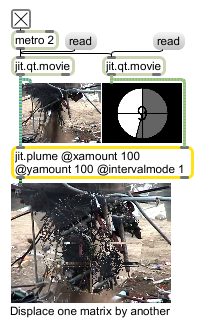# jit.plume

Displace points based on luminance

## Description

The jit.plume object takes two input matrices. The object analyses each incoming cell of the second matrix and, based on the average value of its planes, displaces the corresponding cell in the first matrix.

## Matrix Operator

matrix inputs:2, matrix outputs:1
 Name IOProc Planelink Typelink Dimlink Plane Dim Type in2 resamp 1 1 1 1 1 char out n/a 1 1 1 1 1 char

## Messages

 planeselect plane-list [list] Chooses which planes on the second matrix (inlet 2) are averaged to offset the first matrix. The planeselect message takes the following arguments: -2 = Average all planes -1 = Average all planes except plane 0 (default) 0-x = A single int argument specifies a single plane (no average) A list of int arguments specifies a multiplane average of the planes listed (e.g., 0 2 3)

## Attributes

Name Type g/s Description
intervalmode int Interval mode for x_interval only (default = 0 (entire frame))
0 = interval calculated over the entire frame
1 = new interval count for each scanline
wrap int Wrap mode (default = 0 (normal))
0 = normal: Cells displaced off the edge of the frame are disregarded
1 = wrap: Cells displaced off the edge of the frame wrap to the other edge
2 = reflect: Cells displaced off the edge of the frame are reflected
3 = clip: Cells are clipped to the edge of the frame
x_interval int Equivalent to xinterval.
xamount int The horizontal amount of displacement (multiplied by luminance) (default = 0)
xinterval int The frequency of displacement calculation along the horizontal axis (default = 1)
xoffset int The horizontal offset of displacement (default = 0)
y_interval int Equivalent to xinterval.
yamount int The vertical amount of displacement (multiplied by luminance) (default = 0)
yinterval int The frequency of displacement calculation along the vertical axis (default = 1)
yoffset int The vertical offset of displacement (default = 0)

## Examples